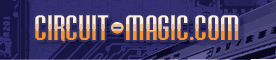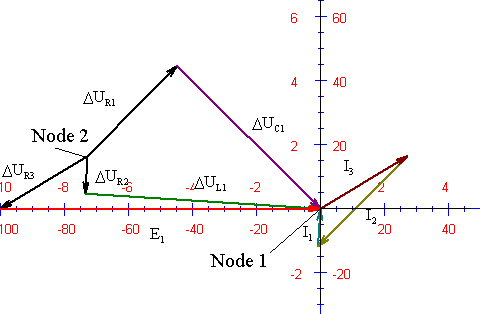Creating Phasor Diagrams with Circuit MagicCircuit analysis Tutorial
AKNM Circuit Magic- circuit analysis software

# Creating Phasor Diagrams with Circuit Magic

Phasor diagram

Step 1. Construct following circuit using Circuit Magic schematics editor.R1=10 ohms; R2=10 ohms; R3=10 ohms;

L1=0,2H; Ń1=200mkF; E1=100 V;

Step 2. Run Node Voltage simulation.

Solution

V1·Y11=I11

Branches impedance calculation

Z1=R2+j·w·L1=10+j·314,2·0,2=10+j62,83

Z2=R1+j·-1/(w·C1)=10+j·-1/(314,2·200·10-6)=10-j15,92

Z3=R3=10=10

Y11=1/Z1+1/Z2+1/Z3=0,1308+j0,02953

I11=-E1/Z3=(-100)/(10)=-10

(0,1308+j0,02953)·j1=-10

j1=-72,76+j16,43

j2=0

I1=(V1-V2)/Z1=0,07524+j1,17

I2=(V1-V2)/Z2=-2,799-j2,813

I3=(V1-V2+E1)/Z3=2,724+j1,643

Step 3. Select Create Phasor diagram Menu item.

Step 5 Change phasors graphics properties.By using phasor diagram above we can easily check solution.

1. Kirchhoff’s Currents Law.

Vector sum of f the currents in all the branches which converge in a common node is equal to zero

(Vector sum of currents phasors I1,I2,I3 is equal to zero)

2. Kirchhoff’s Voltage Law.

Vector sum of the voltages between successive nodes in a closed path in the network is equal to zero.

(Vector sum of voltage phasors in two circuit loops is equal to zero)

Make use of following rules to find mistakes in analysis.

Rule 1.

Phasors of resistance voltage and current through resistance are parallel to each other.

(∆UR1 &I2 , ∆UR3 &I3 , ∆UR2 &I1 ,)

Rule 2.

Phasors of inductance voltage and current through resistance are perpendicular to each other. (Voltage leads current).

Rule 3.

Phasors of capacitance voltage and current through resistance are perpendicular to each other. (Current leads voltaget).